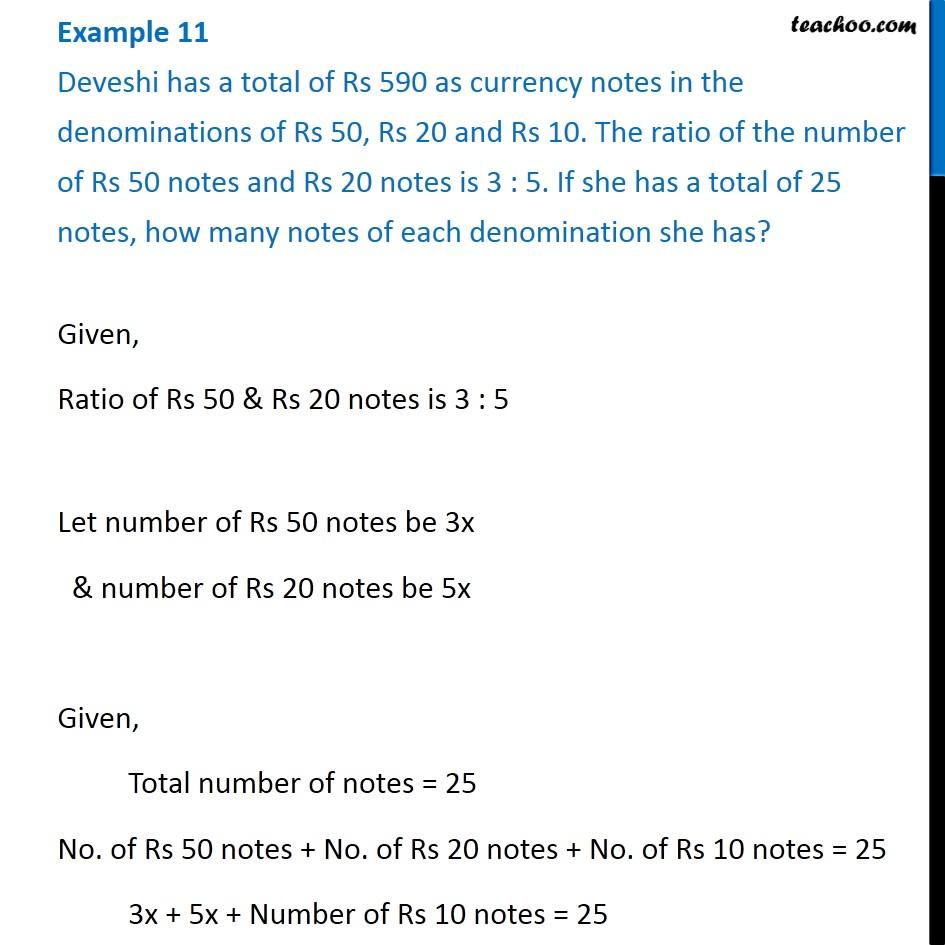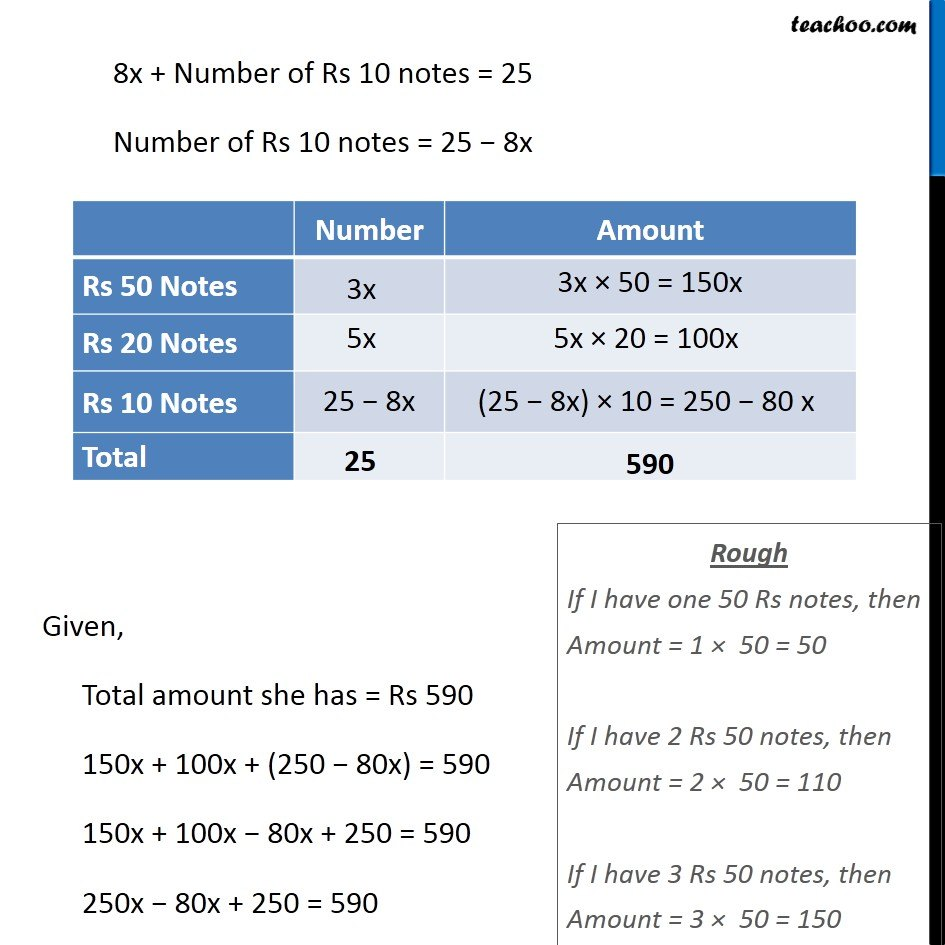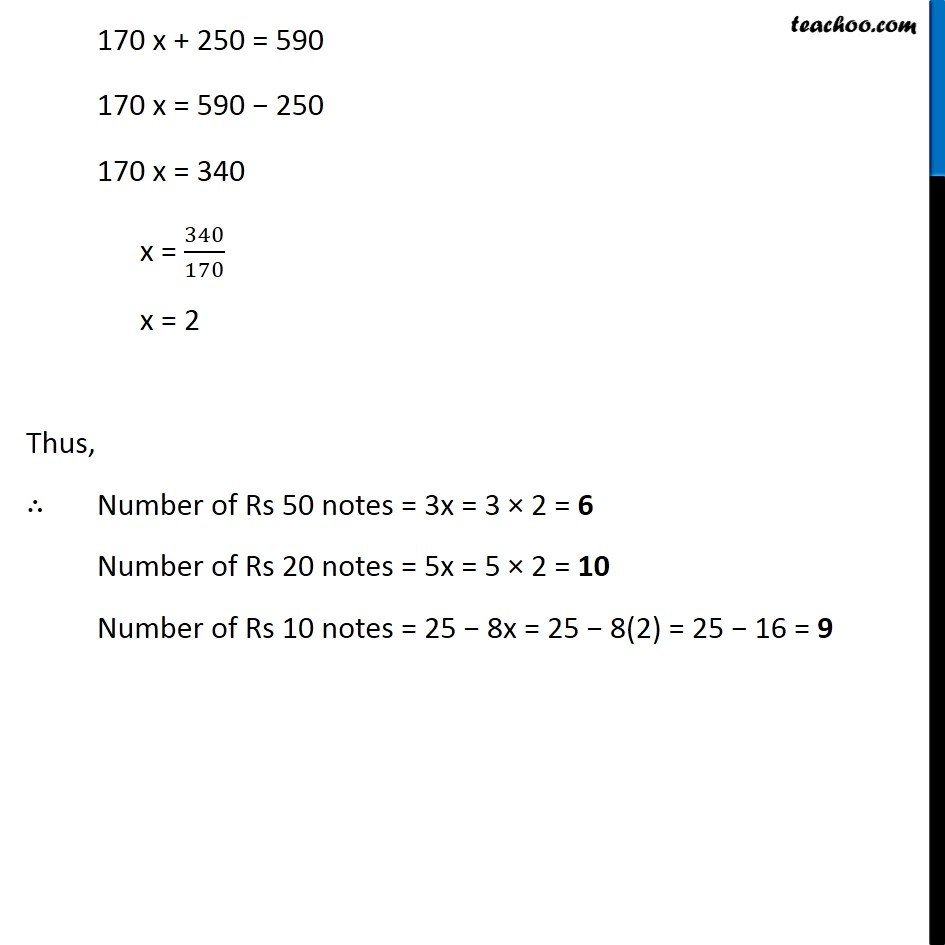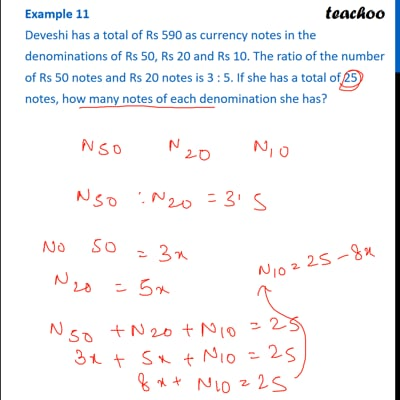Examples

Chapter 2 Class 8 Linear Equations in One Variable
Serial order wiseThis video is only available for Teachoo black users

Get live Maths 1-on-1 Classs - Class 6 to 12

### Transcript

Example 11 Deveshi has a total of Rs 590 as currency notes in the denominations of Rs 50, Rs 20 and Rs 10. The ratio of the number of Rs 50 notes and Rs 20 notes is 3 : 5. If she has a total of 25 notes, how many notes of each denomination she has?Given, Ratio of Rs 50 & Rs 20 notes is 3 : 5 Let number of Rs 50 notes be 3x & number of Rs 20 notes be 5x Given, Total number of notes = 25 No. of Rs 50 notes + No. of Rs 20 notes + No. of Rs 10 notes = 25 3x + 5x + Number of Rs 10 notes = 25 8x + Number of Rs 10 notes = 25 Number of Rs 10 notes = 25 − 8x Given, Total amount she has = Rs 590 150x + 100x + (250 − 80x) = 590 150x + 100x − 80x + 250 = 590 250x − 80x + 250 = 590 Rough If I have one 50 Rs notes, then Amount = 1 × 50 = 50 If I have 2 Rs 50 notes, then Amount = 2 × 50 = 110 If I have 3 Rs 50 notes, then Amount = 3 × 50 = 150 170 x + 250 = 590 170 x = 590 − 250 170 x = 340 x = 340/170 x = 2 Thus, ∴ Number of Rs 50 notes = 3x = 3 × 2 = 6 Number of Rs 20 notes = 5x = 5 × 2 = 10 Number of Rs 10 notes = 25 − 8x = 25 − 8(2) = 25 − 16 = 9